# The Augmented Square Twist

This page describes research done by me (Thomas Hull) and my student, Michael Urbanski during 2017 and 2018. It was presented at the 7OSME conference and appears in an article in the 7OSME Proceedings book. The arxiv version of this paper can be found here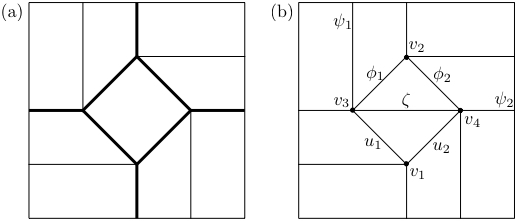The square twist is classic and confounding origami fold that has been studied quite a bit by scientists, mathematicians, and origami artists. Its crease pattern is shown in the above figure (a). If you've never folded this before, I suggest you give it a try! Take a square piece of paper and crease it into 1/4ths horizontally and vertically, to make a 4x4 grid. Then try to crease the "inner diamond" creases shown in the crease pattern. Then make the above folds in Figure (a), where the bold lines are mountain creases and the lighter lines are valley creases. There are also instructions HERE and HERE. To see someone unfolding a square twist, look here.

One aspect of square twists that has been studied quite a bit is the fact that they cannot be folded rigidly. By this we mean that if the panels of paper between the creases were made stiff, like out of sheet metal, and the creases were hinges, then it would not be able to fold up using the mountains and valleys as done in Figure (a) above. (See also here.) Note that if we use different mountain and valley creases, we can make it rigidly fold. One way is shown in the animated gif below. (See this fun article for more information on when twist folds are rigid or not!)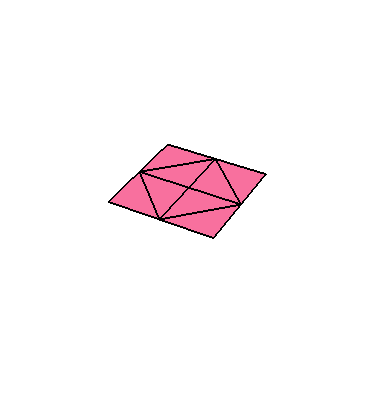But what about the crease pattern in Figure (b) above? We call that the augmented square twist. It is the square twist crease pattern with one crease added to it across the inner diamond, labeled ζ in Figure (b).

Back in 2013, Tomohiro Tachi and I were wondering how we could make the classic square twist of Figure (a) rigidly foldable. Of course, if we just add more creases we could make it rigidly foldable, but we wanted to do it in as simple a way as possible. So we thought of just adding the crease ζ as in Figure (b). The problem was that when trying to fold this crease patten using paper in our own hands, it seemed difficult. It was not obvious how to do make this rigidly fold into a classic square twist pattern, or if it was even possible.

It turned out that it was difficult because this augmented square twist crease pattern takes a circuitous route to rigidly fold. The below animated gif shows how to do it: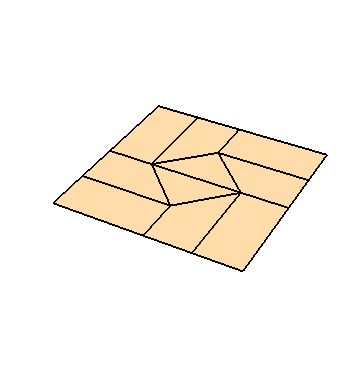How did we discover this? Well, Tomohiro and I just played with physical paper until we stumbled upon this way, and then Tomohiro used his Rigid Origami Simulator to convince ourselves that this was actually a rigid folding. But it wasn't a proof.

And that's where the matter stood for a long while. We could have tried to actually prove that the augmented crease pattern was rigidly foldable, but if you look at it closely, it is made of degree-4 and degree-5 vertices. Degree-4 vertices are very well-understood, with nice, explicit equations that tell us how the folding angles must relate to each other as they rigidly fold and unfold. Degree-5 vertices are not nearly as well-understood. How to make a proof of this wasn't immediately obvious.

So in 2017 I asked a student of mine, Michael Urbanski, a then-sophomore at Western New England University to look at this problem. I challenged him to create Mathematica code that will symbolically model this crease pattern rigidly folding in the way Tomohiro and I predicted it could. After some struggles with Mathematica code and learning how to use rotation matrices to model rigid folding, he succeeded! The result was a Mathematca model that created the above animated gif.

## Other ways to rigidly fold it

But it turned out that Michael's code had some surprises in store for us. Or rather, in order to get this model to work, he had to get Mathematica to solve some complicated trigonometric equations. Some of these equations had more than one solution, and at first we thought they all resulted in degenerate cases that were impossible to fold in real life.

Want to see an example of one? Look below!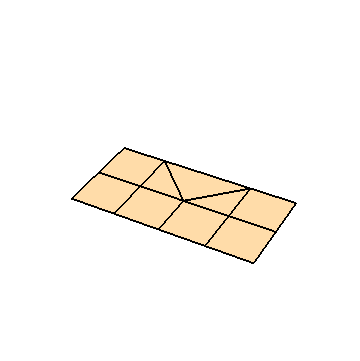Can you tell what is going on there? It is a legitimate way to rigidly fold this augmented square twist crease pattern. Notice how the crease pattern (Figure (b) above) has a horizontal crease going all the way through the paper? Well, we could fold that crease first and then fold the other creases. This forces the paper to self-intersect, as you can see in the above gif. So this isn't something you could do in reality (unless we had phantom paper). But it is a perfectly fine solution to the rigid foldability matrix equations. And you can see how it does fold the paper to the classic square twist pattern.

There's another degenerate case we found: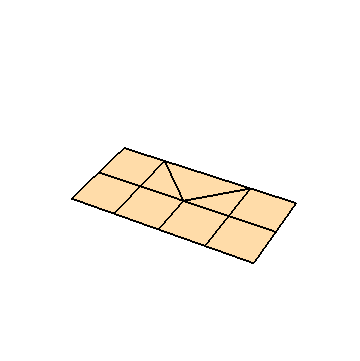That one is similar to the previous degenerate case because it also starts with the paper folded in half. But it then rigidly folds to a different mountain-valley assignment than the classic square twist. Rather, this one is folding to an iso-area square twist, where the creases around the inner diamond alternate mountain-valley-mountain-valley.

Well that was fun, but it did make us wonder if there were other ways to rigidly fold this crease pattern that do not force the paper to self-intersect. And there are! Simply by analyzing the rigid folding angle equations Michael developed, we found the below one: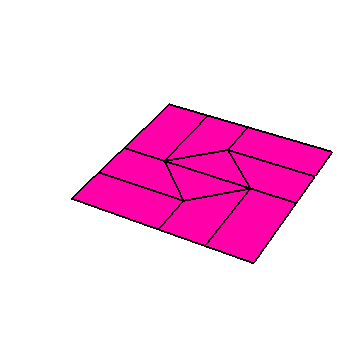Notice that this one starts off by looking like it's going to fold into the iso-area square twist, but then the central create (the ζ one) keeps folding all the way flat, and this causes the paper to intersect itself near the end. So then I thought (inspired by some graphs I was plotting of the folding angle equations) that I could combine this rigid folding with the second, iso-area degenerate one shown previously. Like, literally, I could start folding as shown above, and then switch to the degenerate one after it stopped self intersecting. And it worked!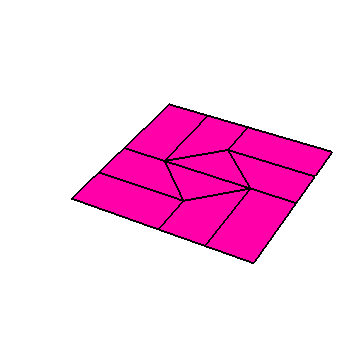This one is a rigid folding of the iso-area square twist. It cannot rigidly fold without the augmenting crease ζ in the middle. This is the same square twist used in Yoshihide Momotani's Stretch Wall model. (See HERE for a video of this model and how to fold it.)

That was cool; we had proven that two nice ways of folding a square twist that are not rigidly foldable can be made to be rigidly foldable if we augment the crease pattern with the ζ crease. But it turned out there was one more way that this augmented crease pattern can rigidly fold. Here it is:This is almost like a combination of the two methods we previously found. Half the paper looks like it's folding like the classic square twist, and the other half looks like it's folding an iso-area square twist. This is literally what is happening. Also notice that in this folding, the paper self-intersects a little bit before it folds flat. There doesn't seem to be a way around that; I tried to do the same trick of combining this rigid folding with one of the degenerate ones, and it does not work. So this is it.

Of course, lots more details can be found in our actual paper.

Want to make these animations on your own, and see animations of the graphs in the paper? Here is the Mathematica code that I used to make these animations and draw the graphs.

These pages Copyright 2018 by Thomas Hull. Last changed 9/13/2018.